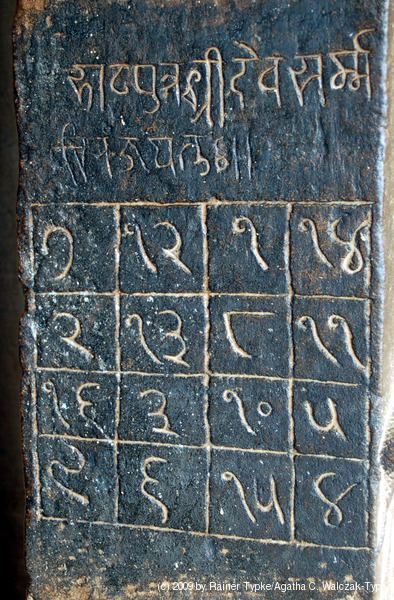# Most perfect magic square

Updated on
Covid-19A most-perfect magic square of order n is a magic square containing the numbers 1 to n2 with two additional properties:

## Contents

1. Each 2×2 subsquare sums to 2s, where s = n2 + 1.
2. All pairs of integers distant n/2 along a (major) diagonal sum to s.

## Examples

Specific examples of most-perfect magic squares that begin with the 2015 date demonstrate how theory and computer science are able to define this group of magic squares.   Only 16 of the 49 2x2 cell blocks that sum to 130 are accented by the different colored fonts in the 8x8 example.

The 12x12 square below was found by making all the 42 principal reversible squares with ReversibleSquares, running Transform1 2All on all 42, making 23040 of each, (of the 23040 x 23040 total each), then making the most-perfect squares from these with ReversibleMost-Perfect. These squares were then scanned for squares with 20,15 in the proper cells for any of the 8 rotations. The 2015 squares all originated with principal reversible square number #31. This square has values that sum to 35 on opposite sides of the vertical midline in the first two rows.

## Physical Properties

The image below shows numbers completely surrounded by larger numbers with a blue background.

## Most-perfect magic square upgraded to a cube

There are 108 of these 2x2 subsquares that have the same sum for the 4x4x4 most-perfect cube.

## Properties

All most-perfect magic squares are panmagic squares.

Apart from the trivial case of the first order square, most-perfect magic squares are all of order 4n. In their book, Kathleen Ollerenshaw and David S. Brée give a method of construction and enumeration of all most-perfect magic squares. They also show that there is a one-to-one correspondence between reversible squares and most-perfect magic squares.

For n = 36, there are about 2.7 × 1044 essentially different most-perfect magic squares.

The second property above implies that each pair of the integers with the same background colour in the 4×4 square below have the same sum, and hence any 2 such pairs sum to the magic constant.

## References

Similar Topics
Ghost Chasers
William King (author)
Gökçek Vederson
Topics